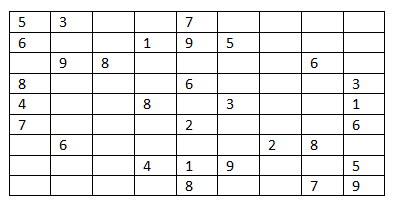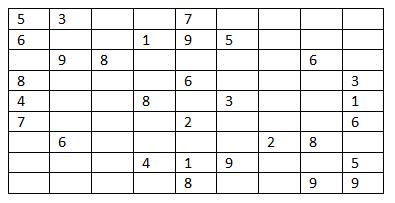Q:

# Check for Valid Sudoku

Determine if a 9x9 Sudoku board is valid. Only the filled cells need to be validated according to the following rules:

1. Each row must contain the digits 1-9 without repetition.
2. Each column must contain the digits 1-9 without repetition.
3. Each of the 9 3x3 sub-boxes of the grid must contain the digits 1-9 without repetition.

Example:

<img src="https://www.includehelp.com/icp/Images/valid-sudoku.jpg" alt="Check for Valid Sudoku" max-width:="" 100%;"="" class="w3-border" style="box-sizing: border-box; border: 1px solid rgb(204, 204, 204) !important; margin-bottom: -5px;">

According to the above three rules - The 9X9 matrix is obviously a valid Sudoku

The above problem can be solved by checking all the three conditions which need to be satisfied for a Sudoku to be valid.

Pre-requisite:

Checking unique numbers in a set:

The above problem can be simply divided to sub-problems where in each sub-problem we simply need to check the numbers in the current set whether all are valid or not.

A current set can consist of numbers from a single row of the Sudoku, or from a single column, or from a 3X3 sub-box.

To check whether all numbers in the set are unique or not we can use set STL

```set<int> s;
For all numbers which are to be included in the set
IF s.find(number)==s.end()
It's a unique number and insert it into the set
ELSE
It's not a unique number
End For
```

Algorithm:

Divide the problem into three sub-problems

1. Check for each row consisting unique number
2. Check for each column consisting unique number
3. Check for each of 9 3X3 sub-boxes consisting unique number

For each sub-problem

1. Declare a set for each row/column/sub-box
2. Check whether the current row/column/sub-box have all unique no or not
3. If any row/column/sub-box fails to have all unique numbers, return false

If there is no such row/column/sub-box that returns false

Then it's a valid Sudoku & return true;

C++ implementation

``````#include <bits/stdc++.h>
using namespace std;

void print(vector<vector<char>> board){
//printing the sudoku
for(auto it=board.begin();it!=board.end();it++){
for(auto ij=(*it).begin();ij!=(*it).end();ij++){
printf("%c ",*ij);
}
printf("\n");
}
}

bool isValidSudoku(vector<vector<char>>& board) {
//checking for row
//check for unique numbers
for(auto it=board.begin();it!=board.end();it++){
set<char> s;
for(auto ij=(*it).begin();ij!=(*it).end();ij++){
if(*ij!='.'){
//finding unique numbers(represented as characters here)
if(s.find(*ij)==s.end()){
s.insert(*ij);
}
else
return false;
}
}
}
//checking for column
//check for unique numbers
for(int i=0;i<9;i++){//9X9 dimension
set<char> s;
for(int j=0;j<9;j++){
if(board[j][i]!='.'){
//finding unique numbers(represented as characters here)
if(s.find(board[j][i])==s.end()){
s.insert(board[j][i]);
}
else
return false;
}
}
}
//checking for sub-boxes
//check for unique numbers
for(int x=0;x<9;x=x+3){
for(int i=0;i<9;i=i+3){
set<char> s;
for(int j=x;j<x+3;j++){
for(int k=i;k<i+3;k++){
//finding unique numbers(represented as characters here)
if(board[j][k]!='.'){
if(s.find(board[j][k])==s.end()){
s.insert(board[j][k]);
}
else
return false;
}
}
}
}
}
return true;
}

int main(){
cout<<"black spaces represented as '.'\n";
vector<vector<char>> a{
{'5','3','.','.','7','.','.','.','.'},
{'6','.','.','1','9','5','.','.','.'},
{'.','9','8','.','.','.','.','6','.'},
{'8','.','.','.','6','.','.','.','3'},
{'4','.','.','8','.','3','.','.','1'},
{'7','.','.','.','2','.','.','.','6'},
{'.','6','.','.','.','.','2','8','.'},
{'.','.','.','4','1','9','.','.','5'},
{'.','.','.','.','8','.','.','7','9'}};

print(a);

if(isValidSudoku(a))
cout<<"It's a valid one"<<endl;
else
cout<<"It's a invalid sudoku\n";

vector<vector<char>> b{
{'5','3','.','.','7','.','.','.','.'},
{'6','.','.','1','9','5','.','.','.'},
{'.','9','8','.','.','.','.','6','.'},
{'8','.','.','.','6','.','.','.','3'},
{'4','.','.','8','.','3','.','.','1'},
{'7','.','.','.','2','.','.','.','6'},
{'.','6','.','.','.','.','2','8','.'},
{'.','.','.','4','1','9','.','.','5'},
{'.','.','.','.','8','.','.','9','9'}};

print(b);

if(isValidSudoku(b))
cout<<"It's a valid one"<<endl;
else
cout<<"It's a invalid sudoku\n";

return 0;
}
``````

Output

```black spaces represented as '.'
5 3 . . 7 . . . .
6 . . 1 9 5 . . .
. 9 8 . . . . 6 .
8 . . . 6 . . . 3
4 . . 8 . 3 . . 1
7 . . . 2 . . . 6
. 6 . . . . 2 8 .
. . . 4 1 9 . . 5
. . . . 8 . . 7 9
It's a valid one
5 3 . . 7 . . . .
6 . . 1 9 5 . . .
. 9 8 . . . . 6 .
8 . . . 6 . . . 3
4 . . 8 . 3 . . 1
7 . . . 2 . . . 6
. 6 . . . . 2 8 .
. . . 4 1 9 . . 5
. . . . 8 . . 9 9
It's a invalid sudoku
```

Comment on outputsFor the first Sudoku, there is no violation of Sudoku rule for each row, each column, each of 9 3X3 sub-boxes. Thus it’s valid Sudoku.For the second one there is two 9 in the last row, which violates the Sudoku rule. Thus it’s an invalid one.